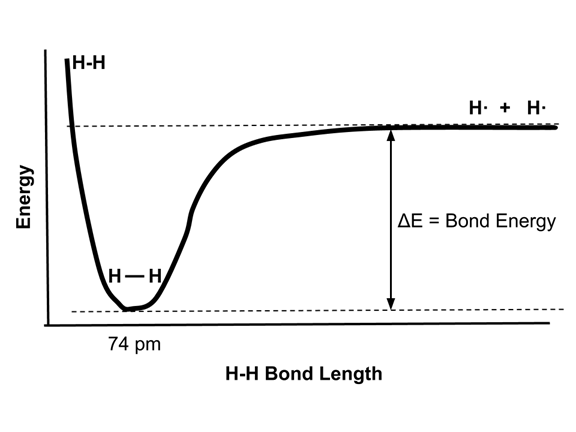# Enduring Understanding 5.C.1: Bond lengths and dissociation energies

• As two atoms approach each other, the electrons of one atom are attracted to the nucleus of the other atom. The potential energy of the system drops.
• When the two atoms get very close, the electron clouds repel each other and the energy of the system increases rapidly.
• The distance at which the energy is minimized is called the bond length. The energy drop of the system from the two separated atoms is the bond energy.
• The bond length and bond energy are illustrated in the following energy diagram of the hydrogen molecule, H2.
•• The bond dissociation energy, ΔE, is the energy released by two isolated hydrogen atoms when they come together to the energy minimum bond distance of 74 pm. This energy is 436 kJ/mol for H2.
• Bond making and bond breaking are opposing processes that involve the same amount of energy. Therefore, the formation of 1 mole of H-H bonds from 2 moles of H atoms releases 436 kJ/mol, and the breaking of 1 mole of H-H bond to form 2 moles of H atoms requires 436 kJ/mol.
• Some typical bond dissociation energies are illustrated here.
• Shorter bonds tend to be stronger than longer bonds.
• Multiple (double) bonds are stronger than single bonds because they involve the sharing of more electron pairs.
• Bond Bond Dissociation Energy (kJ/mol) Notes
Cl-Cl242Chlorine, shorter than Br2 or I2
Br-Br192
I-I151Iodine, very long & weak bond
C-C356Alkane, as in ethane
C=C632Alkene, as in ethylene, double bond
C≅C837Alkyne, as in acetylene, triple bond
N≅N945Dinitrogen, one of the strongest bonds known
• Sample question: Which compound would have a stronger (higher bond dissociation energy) carbon-nitrogen bond, CH3NH2 or CH3CN?
• Acetonitrile, CH3CN, has a triple carbon-nitrogen bond, which would be much stronger and have a higher dissociation energy than methylamine, CH3NH2, which only has a single C-N bond.

 Related Links: Chemistry Chemistry Quizzes AP Chemistry Notes Chemical Reactions and Calorimetry AP Chemistry Quizzes Atoms, Molecules, and Chemical Analysis Quiz Electronic Structure of Atoms Quiz Periodicity, Atomic & Ionic Radii, Atomic Models Quiz Conservation of Matter and Balanced Equations Quiz Gases and Solutions Quiz Intermolecular Forces Quiz Molecular Geometry and VSEPR Quiz Bonding and Solid State Properties Quiz Balanced and Ionic Equations Quiz Chemical Reactions Quiz Redox Reactions and Electrochemistry Quiz Chemical Kinetics and Rates Quiz Reaction Rates and Collisions Quiz Multi-Step Reaction Rates and Catalysis Quiz Energy and Heat Quiz Conservation of Energy and Calorimetry Quiz Bond Energies Quiz Entropy and Gibbs Free Energy Quiz Chemical Equilibrium Quiz Solubility and Acid-Base Equilibrium Quiz

To link to this Bond lengths and dissociation energies page, copy the following code to your site: